#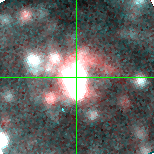M33C-21386 (J013406.80+304727.0)

## Target Notes### Classification: Of/late-WN

(Candidate LBV) Unresolved from LGGS star J013406.75+304727.3 of comparable brightness in all bands within 1 arcsecond. One additional unresolved LGGS star 2 magnitudes fainter within 1 arcsecond. Also a blend of two LGGS stars and a third in addition that are 1 magnitude fainter respectively 2 and 5 arcseconds away.

## Plots of Brightness and Color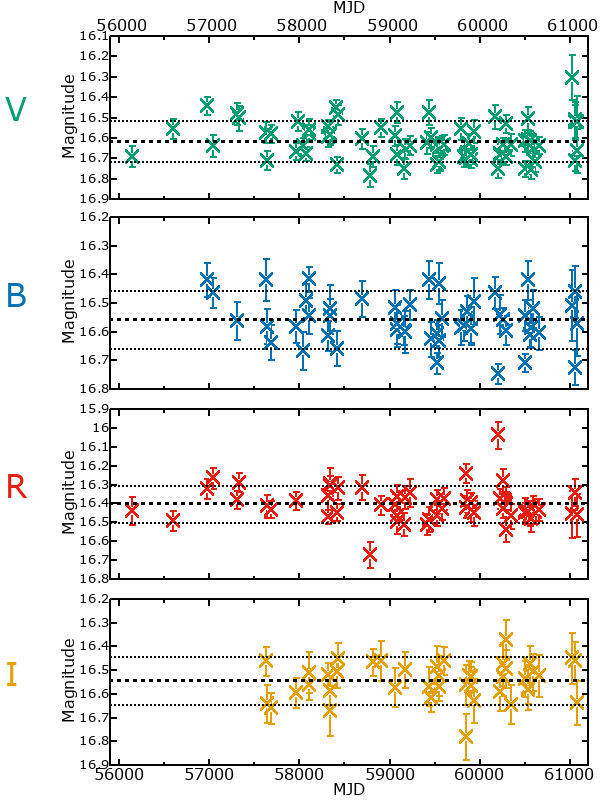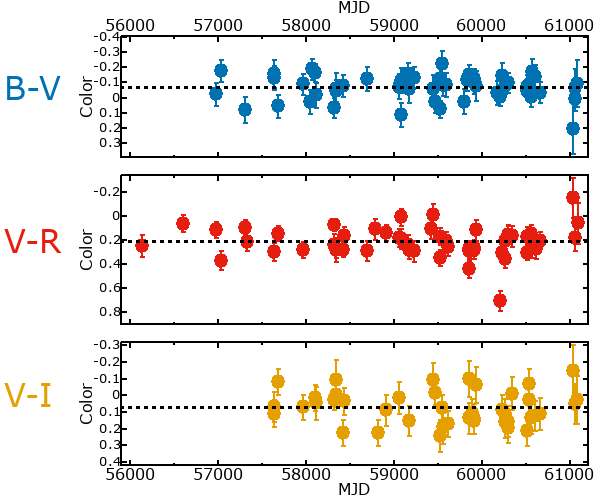## Variability

From the data available in this survey, it is not possible to tell with certainty if this star is variable.

### Correlation factors between bands

If the brightness variation is true variability then there should be a correlation between brightness changes recorded in different filter bands. The following table are the R-squared linear correlation factors calculated for the pairs of bands. The number in parentheses next to the R-squared value is the number of elements used to calculate the correlation factor. The number in square brackets next to the band is the range of magnitudes measured.

V [0.25 mag]B [0.29 mag]R [0.30 mag]
B [0.25 mag]0.21 (22)
R [0.30 mag]0.09 (26)0.28 (19)
I [0.45 mag]0.03 (16)0.10 (12)0.08 (12)

### Magnitude-Magnitude Plots

The plots below are provided to check the values in the table above and also to show any non-linear correlations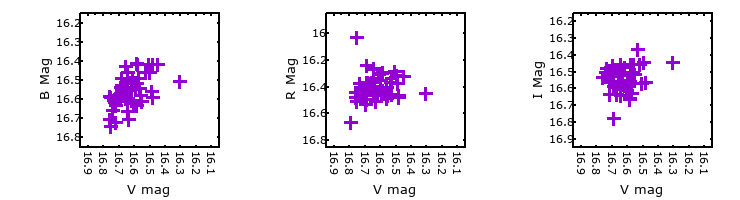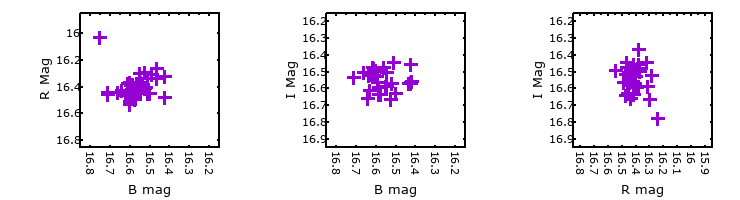### I & R Statistics

Welch & Stetson (1993, AJ, 105, 1813) present a method for varible star detection in CCD images from automated surveys. Data from two bands are compared and two statistics are calculated: R and I.

The I-statistic has an expectation value of zero for stars that are NOT variable. A larger non-zero I-value indicates a higher likelihood that the star is variable. The R statistic indicates how the color of the star changes as the variable grows brighter with R = 1.0 being no change in color.

R and I values have only been calculated if there are more than four (4) matched measurements of brightness in each band.
V
(I|R)
B
(I|R)
R
(I|R)
B
(I|R)
0.88 | 0.79
R
(I|R)
0.70 | 1.090.93 | 0.34
I
(I|R)
0.38 | 1.390.36 | 1.23-0.30 | 0.95